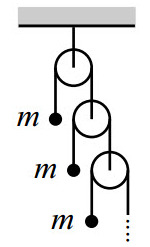# Infinite Atwood SystemConsider a system of infinite pulleys as shown in the figure. Find the acceleration (in $m/s^2$) of the top mass.

Details and Assumptions:
$\bullet$ Initially the masses are held stationary, and then released simultaneously.
$\bullet$ The masses of the pulleys and the strings are negligible.
$\bullet$ $\displaystyle g=9.8m/s^2$

×